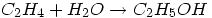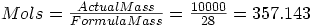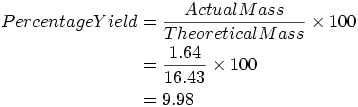## 2006 Q7(c)(iii) 2006 Q7(c)(iii)

### pinklollipop

Posted 26 April 2008 - 03:15 PM

can anyone help with this plz....if 1.64kg of ethanol (relative formula mass = 46) is produced from 10.0kg of ethene (relative formula mass = 28),calculate the percentage yield of ethanol.

### Xeraii

Posted 11 May 2008 - 06:55 PM

First you should write out the equation and balance it in order to find the mole ratio of reactants and products.In this case the ratio is 1 : 1 and the equation is already balanced. Now you have to find the number of mols of reactant used, ie. the number of mols of ethene.In theory, since the ratio is 1 : 1, 357.143 mols of ethene will produce 357.143 mols of ethanol when hydrated. In order to calculate the actual percentage yield you must find the theoretical mass of ethanol produced.

By rearranging the above equation:Now you simply use the equation to calculate percentage yield.